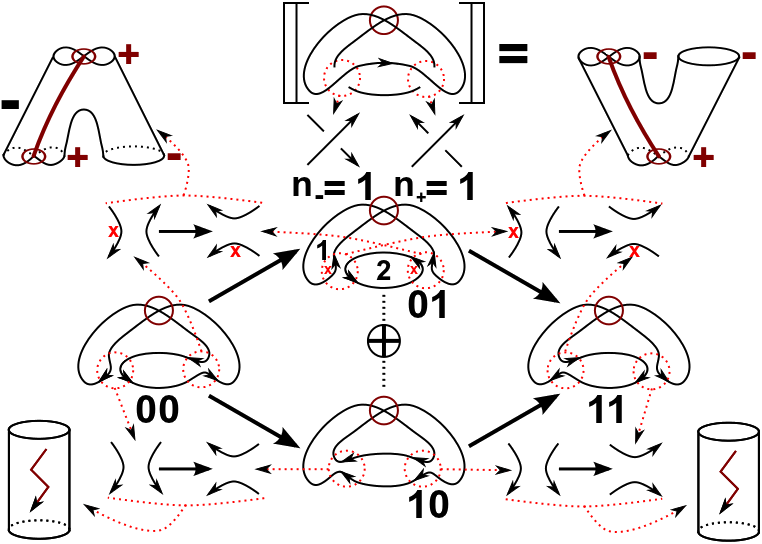## Abstract

We give a geometric interpretation of the Khovanov complex for virtual links. Geometric interpretation means that we use a cobordism structure like Bar-Natan, but we allow non orientable cobordisms. Like Bar-Natans geometric complex our construction should work for virtual tangles too. This geometric complex allows, in contrast to the geometric version of Turaev and Turner, a direct extension of the classical Khovanov complex ($$h=t=0$$) and of the variant of Lee ($$h=0,t=1$$). Furthermore we give a classification of all unoriented TQFTs which can be used to define virtual link homologies with this geometric construction.

## A few extra words

The following picture explains the whole idea. The main ingredient are immersed, possible non-orientable cobordisms with decorated boundary components, i.e. each boundary of an orientable gets a decoration from $$\{+,-\}$$, and a formal sign, which is either $$+1$$ or $$-1$$. All the information can be read of from local data, i.e. from an a priori chosen orientation of the resolutions and an also a priori chosen numbering of the circles in the resolutions. Note that different choices lead to the same complexes modulo chain isomorphisms.
Note that the virtual Khovanov homology is strict stronger then the virtual Jones polynomial, i.e. we show that there are v-knots with seven crossings with equal v-Jones polynomial, but different v-Khovanov homology.